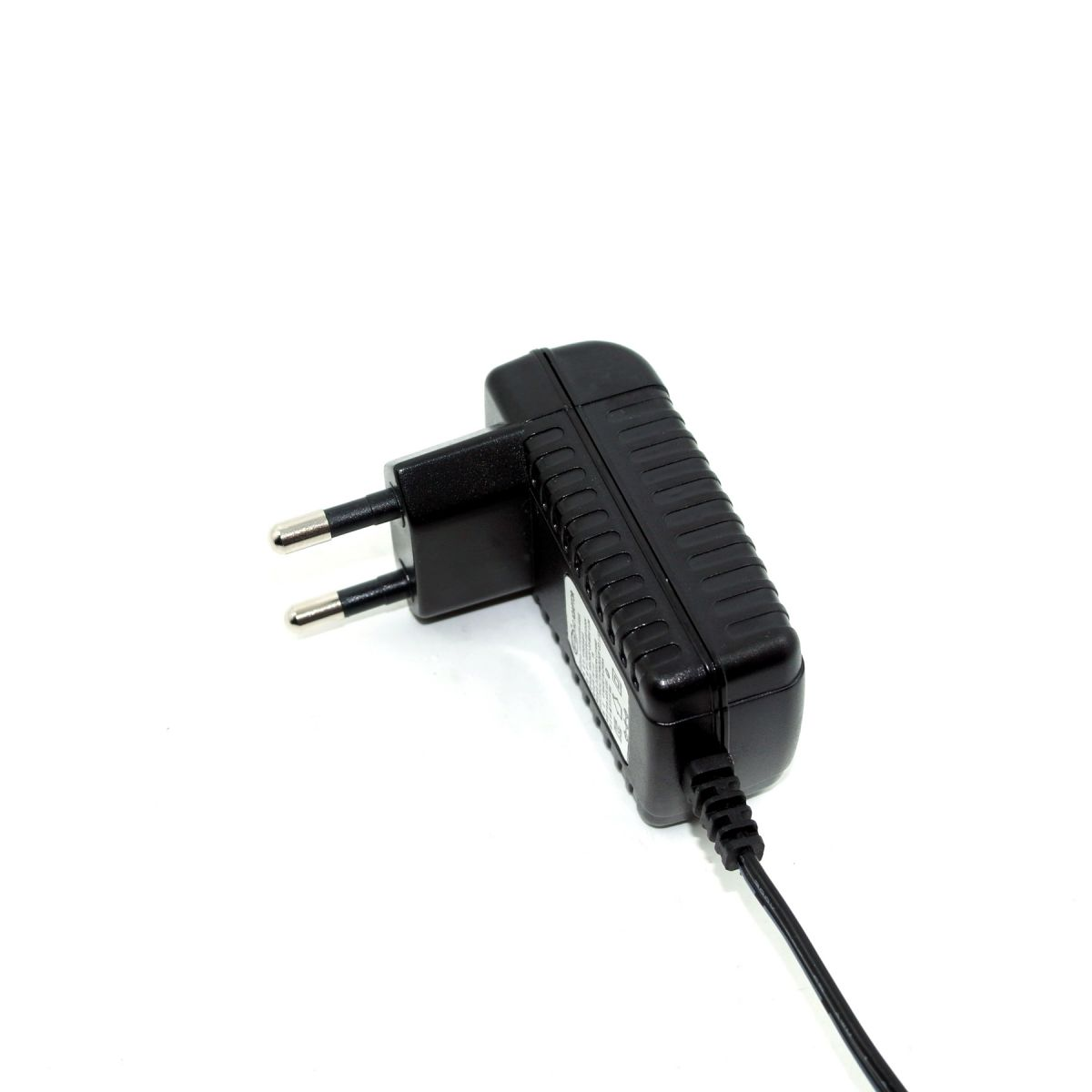Home >> Knowledge >>
Capacitance
Capacitance (or Capacitance, Capacitance)
Refers to the reserves of charge in the context of the given potential difference;Let's call it C, and the international unit is farad (F).Generally speaking, the electric charge will be forced to move in the electric field. When there is a medium between the conductors, it will prevent the charge from moving and make the charge accumulate on the conductor.The most common example of the accumulation of charge is two parallel metal plates.It's also called a capacitor.1. Capacitors are generally represented by "C" plus Numbers in the circuit (for example, C13 represents capacitors numbered 13).Capacitance is made up of two metal films that are separated by an insulating material.The main characteristic of capacitance is the direct current ac.Capacitance refers to the amount of energy that can be stored. The hindering effect of capacitance on ac signals is called capacitive reactance, which is related to the frequency and capacitance of ac signals.Capacitance XC=1/2 PI fc(f for ac signal frequency and C for capacitance capacity) common types of capacitors used in telephones are electrolytic capacitors, ceramic capacitors, patch capacitors, monolithic capacitors, tantalum capacitors, and polyester capacitors.Please visit: power transmission and distribution network for more information

2. Identification methods: the identification methods of capacitance and resistance are basically the same, including direct labeling method, color labeling method and number labeling method.The basic units of capacitance are expressed in farad (F). Other units are: femtofarad (mF), microfarad (uF), nanofarad (nF) and picofarter (pF).Including 1: ferrari = 103 = 106 micro method = 109 = 1012 skin method of large capacity capacitance on capacitance directly to mark its capacity values, such as 10 uf / 16 v capacity small capacitance of the capacity value with letters or Numbers on the capacitance letter notation: 1 m = 1000 uf1p2 = 1.2 PF1n = 1000 pf digital representation: three figures show that the size, is commonly used in the former two significant figures, said the third digit ratio.For example, 102 means 10 102PF=1000PF224, 22 104PF=0.22uF3, and the allowable error of table symbol FGJKLM is 1%, 2%, 5%, 10%, 15%, 20%.

Anna
2019-3-26

Disclaimer: This article comes from individual, KRECO has the right of final interpretation.